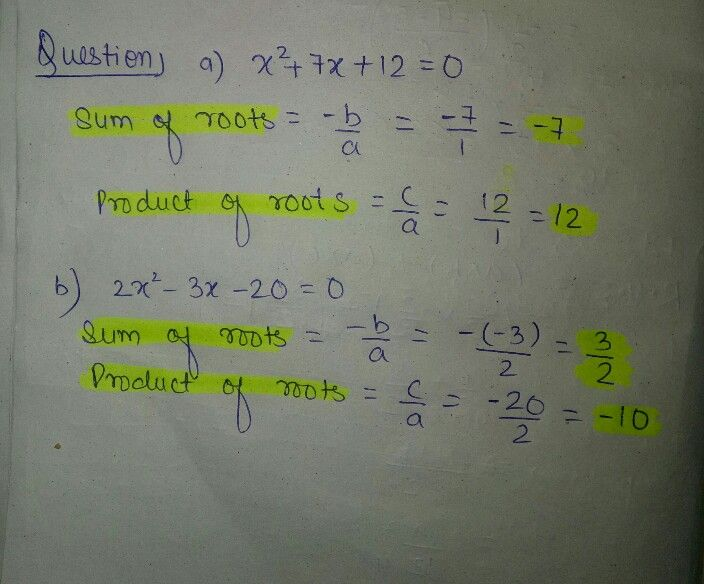Symbol
Problem$3$ $ComP|e+e$ the following $1ab|e:$ Quadratic Equation $110n$ Sum of Roots Product of Roots $x^{2+7x+12}=0$ $2x^{2}-3x-20=0$